Extensions to the Paillier Cryptosystem with Applications to Cryptological Protocols Mads J. Jurik August 2003

### Abstract:

The main contribution of this thesis is a simplification, a generalization and some modifications of the homomorphic cryptosystem proposed by Paillier in 1999, and several cryptological protocols that follow from these changes.

The Paillier cryptosystem is an additive homomorphic cryptosystem, meaning that one can combine ciphertexts into a new ciphertext that is the encryption of the sum of the messages of the original ciphertexts. The cryptosystem uses arithmetic over the group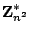and the cryptosystem can encrypt messages from the group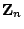. In this thesis the cryptosystem is generalized to work over the group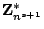for any integer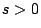with plaintexts from the group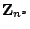. This has the advantage that the ciphertext is only a factor of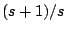longer than the plaintext, which is an improvement to the factor of 2 in the Paillier cryptosystem. The generalized cryptosystem is also simplified in some ways, which results in a threshold decryption that is conceptually simpler than other proposals. Another cryptosystem is also proposed that is length-flexible, i.e. given a fixed public key, the sender can choose the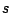when the message is encrypted and use the message space of. This new system is modified using some El Gamal elements to create a cryptosystem that is both length-flexible and has an efficient threshold decryption. This new system has the added feature, that with a globally setup RSA modulus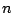, provers can efficiently prove various relations on plaintexts inside ciphertexts made using different public keys. Using these cryptosystems several multi-party protocols are proposed:

• A mix-net, which is a tool for making an unknown random permutation of a list of ciphertext. This makes it a useful tool for achieving anonymity.
• Several voting systems:
• An efficient large scale election system capable of handling large elections with many candidates.
• Client/server trade-offs: 1) a system where vote size is within a constant of the minimal size, and 2) a system where a voter is protected even when voting from a hostile environment (i.e. a Trojan infested computer). Both of these improvements are achieved at the cost of some extra computations at the server side.
• A small scale election with perfect ballot secrecy (i.e. any group of persons only learns what follows directly from their votes and the final result) usable e.g. for board room election.
• A key escrow system, which allows an observer to decrypt any message sent using any public key set up in the defined way. This is achieved even though the servers only store a constant amount of key material.
The last contribution of this thesis is a petition system based on the modified Weil pairing. This system greatly improves the naive implementations using normal signatures from using an order of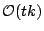group operations to using only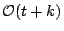, whereis the number of signatures checked, and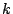is the security parameter.

Available as PostScript, PDF.